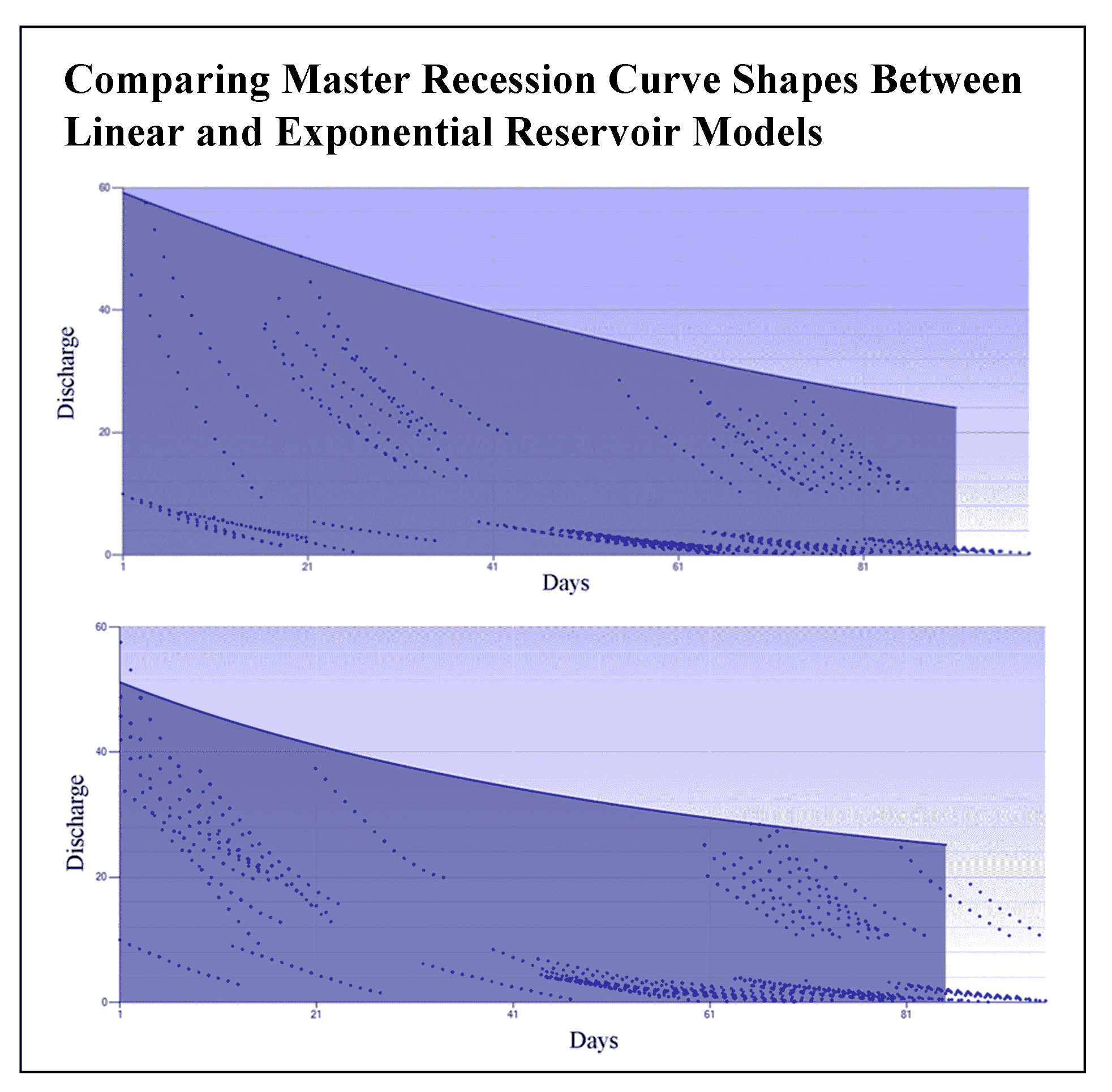Gatha Cognition®
Perception, Learning and Reasoning

#### Comparing Master Recession Curve Shapes Between Linear and Exponential Reservoir Models

6 (2022)

68-72

Exponential Model , Linear , master recession curve , reservoir

##### Altmetric :The behaviour of river flows during periods of recession can be better identified than in other periods. The Master Recession Curve (MRC) approach is a technical approach that is quite effective and efficient in modelling baseflow. This study aims to compare the shape of the MRC between linear and exponential reservoir models. The research method uses two linear reservoir models, the Depuit-Boussinesq equation and an exponential model based on exponential hydraulic conductivity. The results showed that the combination of recession parameters (initial recession discharge, constant and coefficients) for MRC manually linear and exponential reservoir models, and hybridization of genetic algorithm processes, showed that MRC visualization for linear reservoir models was more optimal compared to exponential reservoir models. These results are closely related to the slope of the MRC, where the linear reservoir model is gentler, and the exponential reservoir model is relatively steeper. The slope of the MRC for both reservoir models relates to the storage capabilities of the baseflow and the hydraulic conductivity properties of the study area. The gentle slope of the MRC has the properties of relatively slow storage and is relatively long stored. In contrast, the steep slope of the MRC determines the somewhat wasteful nature of storage.Recession parameterization results show that the linear reservoir model is more optimal than the exponential.

Master Recession Curve (MRC) visualization for linear reservoir models is more gentle than exponential reservoir models.

The slope of the MRC largely determines the flow spool conditions for both models of bottom flow reservoirs.

MRC is one of the most effective and efficient base flow modelling.